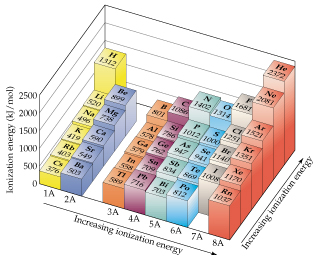# Problem: One way to measure ionization energies is photoelectron spectroscopy (PES), a technique based on the photoelectric effect. In PES, monochromatic light is directed onto a sample, causing electrons to be emitted. The kinetic energy of the emitted electrons is measured. The difference between the energy of the photons and the kinetic energy of the electrons corresponds to the energy needed to remove the electrons (that is, the ionization energy). Suppose that a PES experiment is performed in which mercury vapor is irradiated with ultraviolet light of wavelength 58.4 nm. Write an equation that shows the process corresponding to the first ionization energy of Hg.

###### FREE Expert Solution

We have to write an equation that shows that first ionization energy of mercury.

Ionization energy (I. E.) is the energy required to remove an electron from a gaseous atom or ion.

First I.E. corresponds to removing the first valence electron from a neutral atom.###### Problem Details

One way to measure ionization energies is photoelectron spectroscopy (PES), a technique based on the photoelectric effect. In PES, monochromatic light is directed onto a sample, causing electrons to be emitted. The kinetic energy of the emitted electrons is measured. The difference between the energy of the photons and the kinetic energy of the electrons corresponds to the energy needed to remove the electrons (that is, the ionization energy). Suppose that a PES experiment is performed in which mercury vapor is irradiated with ultraviolet light of wavelength 58.4 nm.Write an equation that shows the process corresponding to the first ionization energy of Hg.